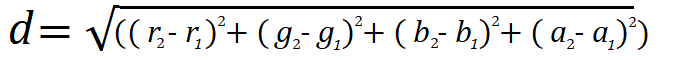# Distance Node

Access: NodesMathDistance

The Distance node outputs the distance between two input vectors using the calculation:The Distance node can be especially useful when calculating the distance between 2D or 3D positions. The output is a single value copied across each component for the vector output, except for the alpha component, which retains its original input value.

# Distance Node Example

 Input Calculation Output InputA = 0.0, 0.0, 0.0, 0.0 InputB = 1.0, 0.0, 0.0, 0.0 d = √((1.0 - 0.0)2 + (0.0 - 0.0)2 + (0.0 - 0.0)2 + (0.0 - 0.0)2) d = 1.0 1.0, 1.0, 1.0, 1.0 InputA = 0.0, 0.0, 0.0, 0.0 InputB = 0.0, 3.0, 4.0, 1.0 d = √((0.0 - 0.0)2 + (3.0 - 0.0)2 + (4.0 - 0.0)2 + (0.0 - 0.0)2) d = 5.0 5.0, 5.0, 5.0, 1.0

Note:  Alpha components retain their original value even if they are included in the calculation.